# Math Practice Topic: Polygon Names

 Description: Classify polygons by their number of sides. Adaptive Learning Progression: Number of sides and/or number of problems increases. Start using MathScore for free

## Sample Levels (out of 6)

Classify the polygons by their number of sides.

 1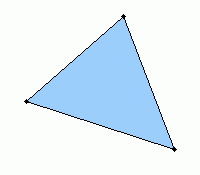triangle quadrilateral pentagon hexagon heptagon octagon nonagon decagon hendecagon dodecagon 2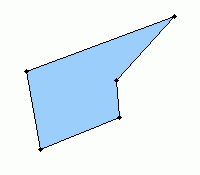triangle quadrilateral pentagon hexagon heptagon octagon nonagon decagon hendecagon dodecagon 3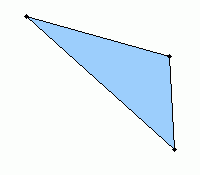triangle quadrilateral pentagon hexagon heptagon octagon nonagon decagon hendecagon dodecagon

Classify the polygons by their number of sides.

 1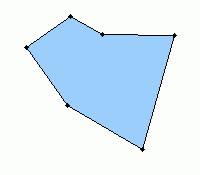triangle quadrilateral pentagon hexagon heptagon octagon nonagon decagon hendecagon dodecagon 2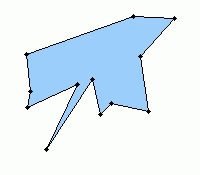triangle quadrilateral pentagon hexagon heptagon octagon nonagon decagon hendecagon dodecagon 3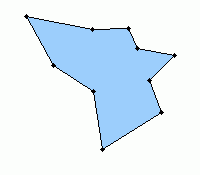triangle quadrilateral pentagon hexagon heptagon octagon nonagon decagon hendecagon dodecagon 4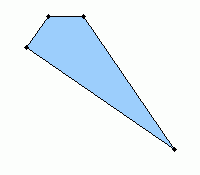triangle quadrilateral pentagon hexagon heptagon octagon nonagon decagon hendecagon dodecagon 5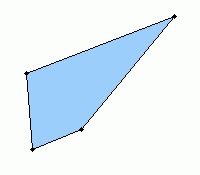triangle quadrilateral pentagon hexagon heptagon octagon nonagon decagon hendecagon dodecagon 6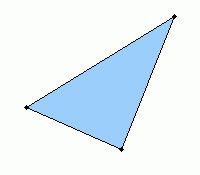triangle quadrilateral pentagon hexagon heptagon octagon nonagon decagon hendecagon dodecagon 7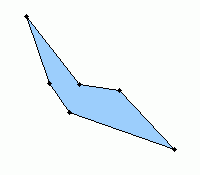triangle quadrilateral pentagon hexagon heptagon octagon nonagon decagon hendecagon dodecagon 8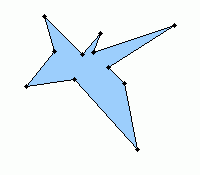triangle quadrilateral pentagon hexagon heptagon octagon nonagon decagon hendecagon dodecagon